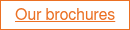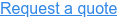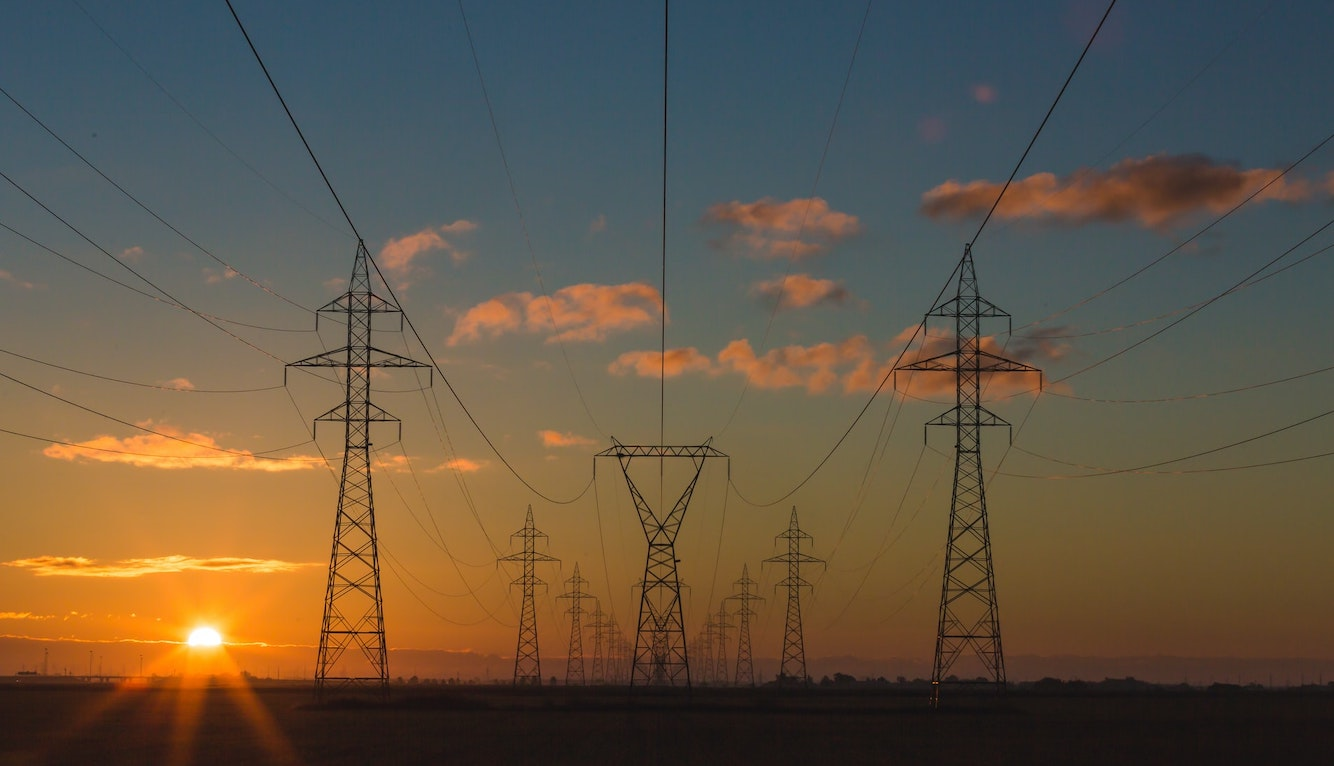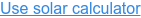Powering Change

Installing since 2010 · 0118 951 4490 · info@spiritenergy.co.uk# Lifetime Cost of Electricity: Solar vs the Grid### Why does lifetime cost matter?

When you purchase a solar PV system, you are essentially buying 25+ years of electricity up front - as this is the expected lifespan of the solar panels. It can therefore be helpful to compare the cost of solar electricity (based on the cost of the system) against the expected cost of grid electricity over that 25 year period. Given historical rises in electricity prices, we can estimate the expected average rates in the future.

### Lifetime cost of solar electricity

We can calculate the cost per unit (kWh) of solar energy by dividing the total electricity generated over 25 years by the combined cost of the system and expected maintenance/inverter replacement cost in that period.

For a typical 4kWp PV system, this gives a lifetime cost of 10p/kWh for solar (you can see the full calculation on our blog).

### Lifetime cost of grid electricity

To calculate the expected average cost of grid electricity over the next 25 years, we look at the Domestic energy price indices published by the government. Over the past 10 years, electricity prices have risen in absolute terms by an average of 4.9% each year. (Adjusting for the ‘GDP deflator’, the average rise is 2.9% each year.)

Though it varies by supplier and part of the country, the current average cost of grid electricity is 34p/kWh.

So, assuming this continues to rise at a rate of 4.9% per year, this gives an average absolute lifetime cost of 64p/kWh for grid electricity over the next 25 years.

Here is how we estimate the price rises each year:

 Year Price per kWh 1 34p 2 35.70p 3 37.40p 4 39.10p 5 41.14p ... ... 23 97.24p 24 102.00p 25 107.10p

### Electricity cost over time

The rise in electricity costs over the past decade has been dramatic:Source: BEIS.

While the cost of renewable technologies has fallen, the cost of generating electricity only makes up a fraction of your bill. Other factors have caused the cost to rise, such as legacy subsidies and maintaining more complex network infrastructure.

### It pays to go solar

The impact is stark when you consider the cost of electricity over your system’s 25 year lifespan: 10p/kWh for solar vs 64p/kWh for the grid.

Looking at it this way, you can see why it pays off to invest in solar energy today - the year-on-year savings only increase as grid costs rise.

For personalised estimates of solar costs and payback, try out our free calculator: